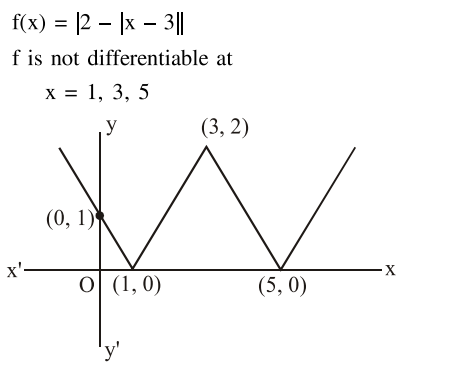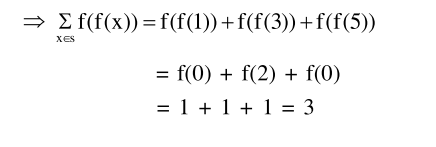# Let S be the set of points where the function,`
Question:

Let $S$ be the set of points where the function, $f(\mathrm{x})=|2-| \mathrm{x}-3||, \mathrm{x} \in \mathrm{R}$, is not differentiable.

Then $\sum_{x \in S} f(f(x))$ is equal to_______.

Solution: